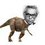# Help required.

Consider a line $$L:3x+4y-10=0$$.

Consider a circle $S_1:x^2+y^2-16x-18y+109=0$.

Find the equation of a certain circle $S_2$ which satisfies the 3 conditions below:

• $S_2$ touches $S_1$ externally.

• $S_2$ touches $L$.

• Line joining the centers of $S_1$ and $S_2$ is perpendicular to $L$.

Do post nice solutions and hints!Note by Nihar Mahajan
6 years ago

This discussion board is a place to discuss our Daily Challenges and the math and science related to those challenges. Explanations are more than just a solution — they should explain the steps and thinking strategies that you used to obtain the solution. Comments should further the discussion of math and science.

When posting on Brilliant:

• Use the emojis to react to an explanation, whether you're congratulating a job well done , or just really confused .
• Ask specific questions about the challenge or the steps in somebody's explanation. Well-posed questions can add a lot to the discussion, but posting "I don't understand!" doesn't help anyone.
• Try to contribute something new to the discussion, whether it is an extension, generalization or other idea related to the challenge.
• Stay on topic — we're all here to learn more about math and science, not to hear about your favorite get-rich-quick scheme or current world events.

MarkdownAppears as
*italics* or _italics_ italics
**bold** or __bold__ bold
- bulleted- list
• bulleted
• list
1. numbered2. list
1. numbered
2. list
Note: you must add a full line of space before and after lists for them to show up correctly
paragraph 1paragraph 2

paragraph 1

paragraph 2

[example link](https://brilliant.org)example link
> This is a quote
This is a quote
    # I indented these lines
# 4 spaces, and now they show
# up as a code block.

print "hello world"
# I indented these lines
# 4 spaces, and now they show
# up as a code block.

print "hello world"
MathAppears as
Remember to wrap math in $$ ... $$ or $ ... $ to ensure proper formatting.
2 \times 3 $2 \times 3$
2^{34} $2^{34}$
a_{i-1} $a_{i-1}$
\frac{2}{3} $\frac{2}{3}$
\sqrt{2} $\sqrt{2}$
\sum_{i=1}^3 $\sum_{i=1}^3$
\sin \theta $\sin \theta$
\boxed{123} $\boxed{123}$

## Comments

Sort by:

Top Newest

Log in to reply

The method i suggest is that, the common tangent of the circles touching externally is the radical axis.

let $S_{2}=x^2+y^2+2gx+2fy+c=0$

Given $S_{1}=x^2+y^2-16x-18y+109=0$

Radical axis of these circle is $L$

$S_{1}-S_{2}=L$

compare the coefficient of $x$ and $y$ and constant.

Hope it helps

- 6 years ago

Log in to reply

actually the conditions u have put up are satisfied by the radical axis of circles

- 6 years ago

Log in to reply

Actually if you calculate out then it will come out that L does not touch S1 so it can not be the radical axis for the two touching circles.

- 6 years ago

Log in to reply

I had the same doubt. Tanishq bhayya wants to say that if we "construct" the radical axis and compare it with $L$ we get the requirements for equation of required circle.

- 6 years ago

Log in to reply

I tried to post my solution. I am having problem with the image uploading.

- 6 years ago

Log in to reply

What problem are you having with uploading the pic?

- 6 years ago

Log in to reply

Can u make a figure to justify ur statement, well i will post mine in few minutes

- 6 years ago

Log in to reply

Sorry to say but it is not necessary that $S_1$ touches $L$. :(

- 6 years ago

Log in to reply

but radical axis is a line perpendicular to the line joining their centres

- 6 years ago

Log in to reply

Oh! I get it. So the strategy is to construct a radical axis , and since the slopes are equal , we can get the radius of required circle by comparing the equation of the lines.

- 6 years ago

Log in to reply

I think satvik's way is easier. While solving by your method , I encountered difficulties in computing $c$ which gave rise to a huge quadratic and I was f**ked! Anyway , I appreciate this idea of radical axis.

- 6 years ago

Log in to reply

The equation of S1 can be rewritten as $(x-8)^{2}+(y-9)^{2}=(6)^{2}$

The distance of the line L from center of S1 is

$\frac {3 (8)+4 (9)-10}{\sqrt {3^{2}+4^2}}=10$

And since the radius of S1 is 6 The minimum distance between the S1 and the line is 4 which can also be seen as the diameter of the touching circle S2. Therefore the radius of S2 is 2. Now we have to find its centre.

The slope of the line perpendicular to L which is also the line joining their centers is 4/3.

Now using the parametric form

$\frac {x-8}{3/5}=\frac {y-9}{4/5}=-8$

Since the distance between the two centers is (6+2)=8 and - sign can be clear from the diagram.

x=16/5 y=13/5 the coordinates of the center of S2.

Equation is

$(x-16/5)^{2}+(y-13/5)^{2}=(2)^{2}.$

I might have done several mistakes please notify me if you find any. Hope I am right?

- 6 years ago

Log in to reply

Absolutely correct!

Alternate way : Find intersection of $L$ and the line joining centers. And use section formula with ratio $4:1$ to obtain center.I obtained radius in the same way as yours.

Thanks @satvik choudhary !

- 6 years ago

Log in to reply

How to upload images i was just able to provide a link to it.

- 6 years ago

Log in to reply

!<no space>[<title>]<no space>(<link>)

Don't type: no space , title , link

- 6 years ago

Log in to reply

- 6 years ago

Log in to reply

×

Problem Loading...

Note Loading...

Set Loading...